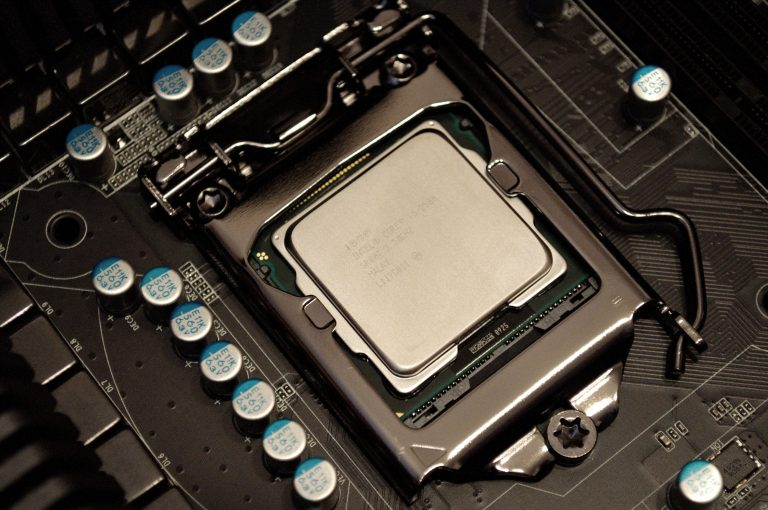Want to share your content on R-bloggers? click here if you have a blog, or here if you don't.I recently purchased a new laptop with an Intel i7-8750 6 core CPU with multi-threading meaning I have 12 logical processes at my disposal. Seemed like a good opportunity to try out some parallel processing packages in R. There are a few packages in R for the job with the most popular being `parallel`, `doParallel` and `foreach` package.

First we need a good function that puts some load on the CPU. We’ll use the Boston data set, fit a regression model and calculate the MSE. This will be done 10,000 times.

```# data
data(Boston)

# function - calculate the mse from a model fit on bootstrapped samples from the Boston dataset
model.mse <- function(x) {
id <- sample(1:nrow(Boston), 200, replace = T)
mod <- lm(medv ~ ., data = Boston[id,])
mse <- mean((fitted.values(mod) - Boston\$medv[id])^2)
return(mse)
}

# data set
#x.list <- lapply(1:2e4, function(x) rnorm(1e3, 0, 200))
x.list <- sapply(1:10000, list)

# detect the number of cores
n.cores <- detectCores()
n.cores```

`##  12`

Parallelising computation on a local machine in R works by creating an R instance on each of the cluster members specified by the user, in my case 12. This means each instance needs to have the same data, packages and functions to do the calculations. The function `clusterExport` copies the data frames, loads packages and other functions to each of the cluster members. This is where some thought is needed as to whether or not parallelising computation on will actually be beneficial. If the data frame is huge making 12 copies and storing them in memory will create a huge overhead and may not speed up the computation. For these examples we need to export the Boston data set to cluster. Since the data set is only 0.1 Mb this won’t be a problem. At the end of the processing it is important to remember to close the cluster with `stopCluster`.

## parLapply

Using `parLapply` from the `parallel` package is the easiest way to parallelise computation since `lapply` is simply switched with `parLapply` and tell it the cluster setup. This is my go to function since it is very simple to parallelise existing code. Firstly we’ll establish a baseline using the standard `lapply` function and then compare it to `parLapply`.

```# single core
system.time(a <- lapply(x.list, model.mse))```

```##    user  system elapsed
##   14.58    0.00   14.66```

```# 12 cores
system.time({
clust <- makeCluster(n.cores)
clusterExport(clust, "Boston")
a <- parLapply(clust, x.list, model.mse)})```

```##    user  system elapsed
##    0.03    0.02    4.33```

`stopCluster(clust)`

Much faster than the standard `lapply`. Another simple function is `mclapply` which works really well and even simpler than `parLapply` however this isn’t supported by Windows machines so not tested here.

## parSapply

`parSapply` works in the same way as `parLapply`.

```# sigle core
system.time(a <- sapply(1:1e4, model.mse))```

```##    user  system elapsed
##   14.42    0.00   14.45```

```# 12 cores
system.time({
clust <- makeCluster(n.cores)
clusterExport(clust, "Boston")
a <- parSapply(clust, 1:1e4, model.mse)})```

```##    user  system elapsed
##    0.02    0.05    4.31```

`stopCluster(clust)`

Again, much faster.

## parApply

For completeness we’ll also test the `parApply` function which again works as above. The data will be converted to a matrix for this to be suitable.

```# convert to mar
x.mat <- matrix(1:1e4, ncol = 1)

# sigle core
system.time(a <- apply(x.mat, 1, model.mse))```

```##    user  system elapsed
##   14.27    0.00   14.32```

```# 12 cores
system.time({
clust <- makeCluster(n.cores)
clusterExport(clust, "Boston")
a <- parApply(clust, x.mat, 1, model.mse)})```

```##    user  system elapsed
##    0.00    0.03    4.30```

`stopCluster(clust)`

As expected the parallel version is again faster.

## foreach

The `foreach` function works in a similar way to for loops. If the apply functions aren’t suitable and you need to use a for loop, `foreach` should do the job. Basically what you would ordinarily put within the for loop you put after the `%dopar%` operator. There are a couple of other things to note here,

1. We register the cluster using `registerDoParallel` from the `doParallel` package.
2. Need to specify how to combine the results after computation with `.combine`.
3. Need to specify `.multicombine = TRUE` for multiple parameter returns such as `cbind` or `rbind.`

There are a few other useful parameters for more complex processors however these are the key things.

```# for
system.time({
model.mse.output <- rep(NA, 1e4)
for(k in 1:1e4){
model.mse.output[k] <- model.mse()
}})```

```##    user  system elapsed
##   14.23    0.00   14.23```

```# foreach
system.time({
registerDoParallel(n.cores)
foreach(k = 1:1e4, .combine = c) %dopar% model.mse()
})```

```##    user  system elapsed
##    3.50    0.59    6.53```

`stopImplicitCluster()`

Interestingly foreach is slower than the `parXapply` functions.

## Summary

There are a number of resources on the parallel computation in R but this is enough to get anyone started. If you are familiar with using the apply functions parallelising computations is straight forward but it’s important to keep in mind whether or not that process needs to be executed in parallel.

The post Simple Parallel Processing in R appeared first on Daniel Oehm | Gradient Descending.